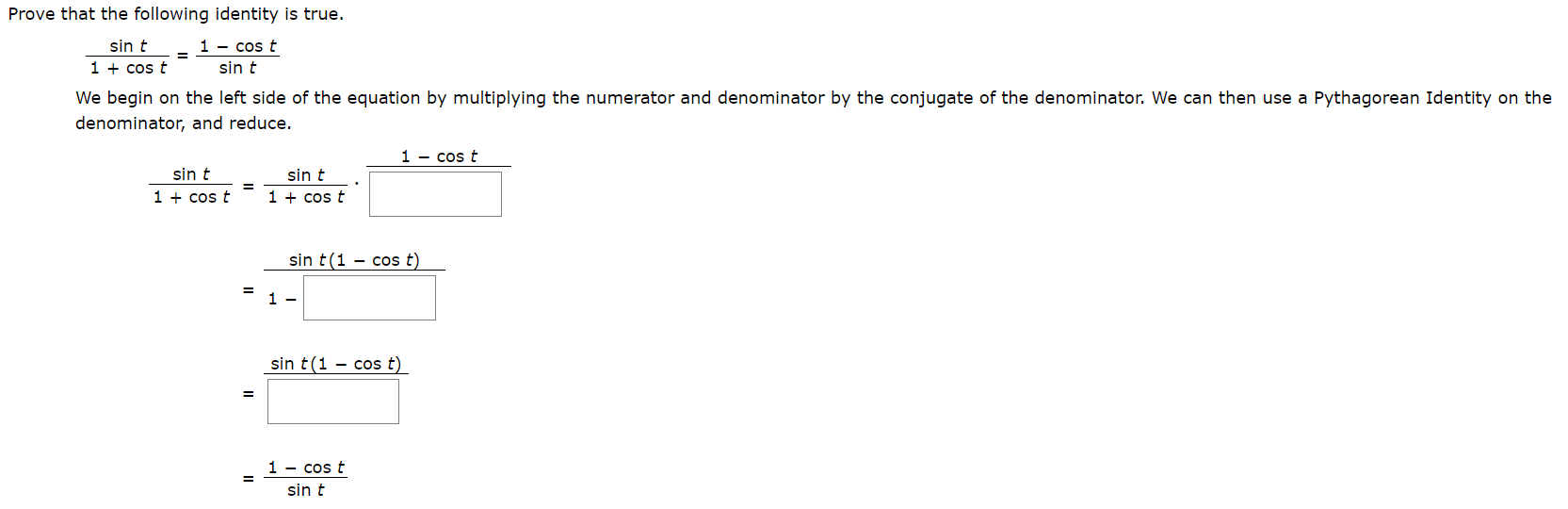# Prove that the following identity is true.sin t1 - cos tsin t1 +cos tWe begin on the left side of the equation by multiplying the numerator and denominator by the conjugate of the denominator. We can then use a Pythagorean Identity on thedenominator, and reduce.1 cos tsin tsin t1 cos t1 cos tsin t(1 - cos t)1 -sin t (1 cos t)1 - cos tsin t

Question
1 views

photo attachedhelp_outlineImage TranscriptioncloseProve that the following identity is true. sin t 1 - cos t sin t 1 +cos t We begin on the left side of the equation by multiplying the numerator and denominator by the conjugate of the denominator. We can then use a Pythagorean Identity on the denominator, and reduce. 1 cos t sin t sin t 1 cos t 1 cos t sin t(1 - cos t) 1 - sin t (1 cos t) 1 - cos t sin t fullscreen
check_circle

Step 1

The given iden...

### Want to see the full answer?

See Solution

#### Want to see this answer and more?

Solutions are written by subject experts who are available 24/7. Questions are typically answered within 1 hour.*

See Solution
*Response times may vary by subject and question.
Tagged in